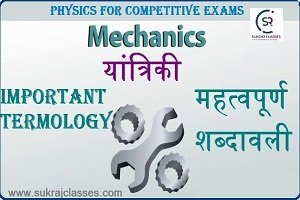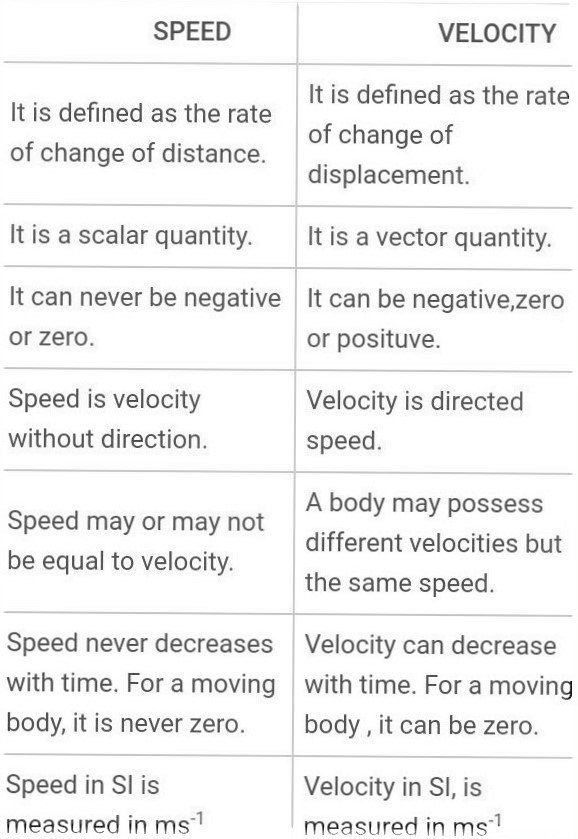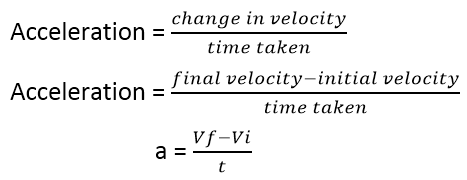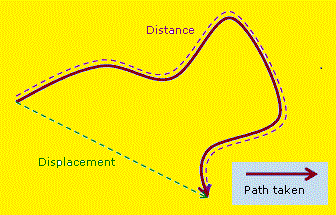# Mechanics Important Terms (यांत्रिकी-महत्वपूर्ण शब्दावली) – Physics Topic

Physics topic – Mechanics Important Terms (यांत्रिकी-महत्वपूर्ण शब्दावली), is important for all competitive exams like SSC CGL, SSC CHSL, RRB NTPC, UPSC and other state civil services exams. In these exams, almost 4-5 questions are coming from Physics. Let’s start topic – Mechanics Important Terms (यांत्रिकी-महत्वपूर्ण शब्दावली):

Mechanics – Important Terms

(यांत्रिकी – महत्वपूर्ण शब्दावली)

MECHANICS

• Mechanics is one of the main branches of physics which deals with the study and behaviour of physical bodies when subjected to different types of forces or displacement, and the subsequent effect of bodies on the environment.
• The action or process of moving or being moved is called motion.

Important Terms in Mechanics:

Speed:-

• Speed is the distance covered by an object in a given amount of time, to that time. It is measured in metres per second.
Speed is the scalar Quantity.
• SI unit of speed is m/s.
• Formula: – speed = distance/time

S      =    d / t

Velocity:

• Velocity is defined as “The rate of displacement of a body is called its velocity.”
• The velocity tells us not only the speed of a body but also the direction along which the body is moving.
• The velocity of a body is a vector quantity.
• Represented as:

Velocity = displacement/time
V =  d/t

• SI unit of velocity is the same as speed i.e. meter per second.

Difference between speed and velocity:-Mechanics Important Terms (यांत्रिकी-महत्वपूर्ण शब्दावली)

Acceleration: –

• The rate of change of the velocity of a body with respect to time.
• Accelerations are the vector quantities.
• The acceleration unit is the meter per Second Square (m/s²).
• Positive acceleration and negative acceleration are two types of acceleration.
• Acceleration formula:Where, a as an acceleration, initial velocity as Vi , final velocity as Vf and t is the time interval, SI unit of acceleration is meter per second per second ms-2

Distance: –

• Distance is defined as the amount of space between two things or the state of being far apart.Distance Formula:

D =  d1 + d2

Displacement: –

• Displacement is defined to be the change in position of an object.
• Displacement is a vector. This means it has a direction as well as a magnitude.

For More:

Force:-

• A force moves or tends to move stops or tends to stop the motion of the object. The force can also change the direction of the motion of an object. It can also change the shape or size of a body on which it acts.
• It is a vector quantity it has magnitude as well as direction.
• The SI unit of force is Newton denoted by N.1 N = 1 kgms-2

Pressure: –

Pressure is the force acting normally per unit area on the surface of a body.

• It is a scalar quantity.
• Its SI unit is Pascal, denoted by “pa”.
• It is measured by Mono-meter.

Mass:-

• Mass as the measure of the amount of matter in a body.
• The SI unit of mass is Kilogram (kg).

Weight: – Weight is the force exerted on a body by gravity.

• Expressed in the formula W = mg,
• Where, W is the weight, m the mass of the object, and g gravitational acceleration.
• The value of weight changes on earth, moon, mars etc…
• SI unit: Newton(N)

Difference between Mass and Weight:-

 Mass Weight Mass can never be zero. Weight can be zero. As in space if no gravity acts upon an object, its weight becomes zero. Mass is a scalar quantity. It has magnitude. Weight is a vector quantity. It has magnitude and is directed toward the centre of the Earth or other gravity well. Mass is commonly measured in kilograms and grams. Weight is commonly measured in Newton. Mass doesn’t change according to location. Weight varies according to location. The mass may be measured using an ordinary balance. Weight is measured using a spring balance.

Mechanics Important Terms (यांत्रिकी-महत्वपूर्ण शब्दावली)

Work:-

Work measure of energy transfer that occurs when an object is moved over a distance by an external force at least part of which is applied in the direction of the displacement.

Energy:-

• Energy is a quantitative property that must be transferred to an object in order for it to perform work. Hence we can define energy as the strength to do any kind of physical activity.
• Energy is essential to life and all living organisms. The sun, directly or indirectly, is the source of all the energy available on Earth